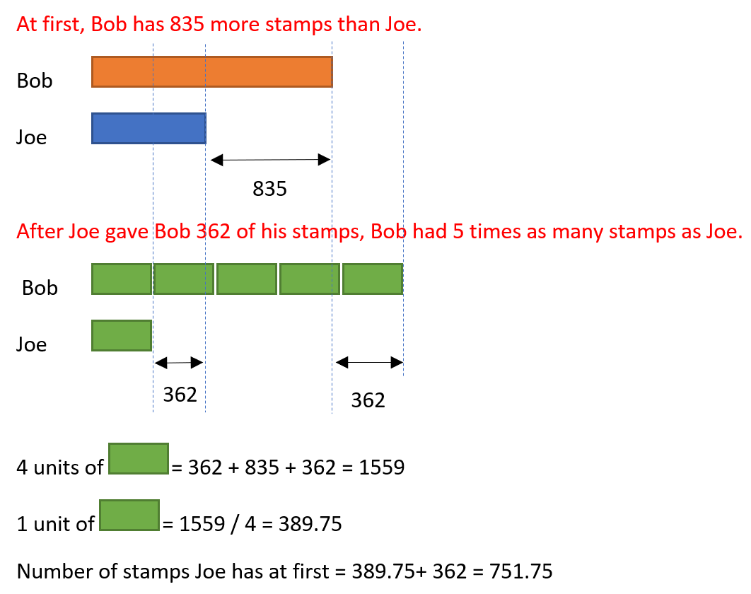# Answer

To correct a flaw in the original question, I have made a minor change, that is ‘835’ to ‘836’, and solved it as follows :

Bob had 836 more stamps than Joe. After Joe gave Bob 362 of his stamps, Bob had 5 times as stamps as Joe. how many stamps did Joe have at first?

Bob : 1 unit + 362 + 836
Joe : 1 unit + 362

4 units = 362 + 836 + 362 = 1560
1 unit = 1560/4 = 390
390 + 362 = 752

Ans : 752 stamps.

0 Replies 0 Likes0 Replies 0 Likes

This is a Before After question.

 Before Transfer After Bob 1u + 835 + 362 1u + 1197 Joe 1u – 362 1u – 362

(1u + 1197) / 5 = (1u – 362) / 1

1u + 1197 = 5u – 1810

1197 + 1810 = 5u – 1u

4u = 2007

1u = 3007 ÷ 4 = 751.75

Since the stamps must be a whole number, this is not possible.

It could be the figure 835 or 362 or 5 times is wrong.

Alternate way

835 + 362 + 362 = 1559

1559 ÷ 4 = 389.75

389.75 + 362 = 751.75

0 Replies 0 Likes